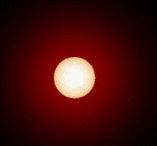## Research Papers in Physics and Astronomy## Norman R. Simon Papers

1986

Article

#### Citation

Bulletin of the American Astronomical Society, Vol. 18 (1986), p.964.

Copyright 1986 American Astronomical Society. Provided by the NASA Astrophysics Data System

#### Abstract

Consider a radially pulsating star. Expand the radius variation in a Fourier series: R = RO+Aicos(iwt+ϕi) [summation convention]. The velocity is v=--iwAisin(iwt+ϕi). The ratio of the radii at two phases of the cycle may be written

(R1/R2)2 = (L1 T24) / (L2 T14)/(L2 T14) = α

Setting R = Ro + Δr (Δr/Ro « 1), we obtain Ro = 2(αΔr2 - rΔl ) / (1-α). The radial excursion Δr at any phase of the pulsation may be determined from a Fourier fit to the observed velocity curve. Assuming highly accurate velocity data (e.g. CORAVEL data), this can be done very precisely. If we choose phase 2 to be that for which Δr2 = O, we have

α = (2/Ro) Δr1 + 1.

COinS